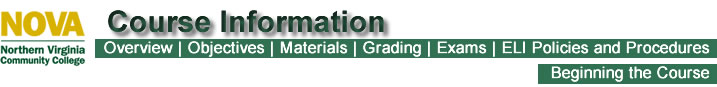PHYSICS 232 - UNIVERSITY PHYSICS II (5 credits)
Overview

This course teaches fundamental principles of physics. It covers mechanics, thermodynamics, wave phenomena, electricity , optics, magnetism, and selected topics in modern physics.

Top
Objectives

The main objective of this course is to learn the principles of physics, including
electricity, magnetism, optics, and wave physics.

Specifically, you will learn to
* calculate electric forces and electric fields from charges.
* calculate electric potential from charge distributions or from the electric field.
* calculate capacitance and resistance of arrangements of conducting materials.
* use Kirchoff’s Laws to find characteristics of dc circuits.
* calculate magnetic forces and magnetic fields.
* understand magnetic induction and to use Lenz’s and Faraday’s Laws.
* calculate characteristics of ac circuits.
* understand the nature of electromagnetic waves and light, as described by Maxwell’s Equations.
*understand phenomena and laws of geometric and wave optics, such as reflection, refraction, polarization, interference and diffraction
* calculate effects in geometric and wave optics, including interaction of light with optical elements such as lenses, mirrors, diffraction gratings.
* describe traveling and standing wave patterns of mechanical waves, understand  and calculate sound effects such as intensity, loudness, pitch, beats, Doppler effect.

In the laboratory you will learn to:
* take accurate measurements with confidence and understand the uncertainties
associated with them and uncertainty propagation, calculate percent error and percent difference
* use computer-assisted laboratory methods, based on ScienceWorkshop interface
* analyze data using graphical and computer-based techniques.
* spread-sheet style analysis of data and computer plots
* analyze data to induce scientific conclusions
* write meaningful conclusions based on observations, calculations, and
data analysis
* learn to collaborate with others as a team to produce collective results
* construct simple circuits with resistors, capacitors, and inductors, using a breadboard

Top
Materials

Top

Your course grade will be determined according to the following percentages.

 Assignment Percentage of Grade 4 Exams (15%each) 60% 7 Video Analysis Lab Reports 20% Homework 20%

No exam, lab, or homework grades will be dropped.

Your final grade will be based on the following scale.

 Grade Percent A 90-100 B 80-89 C 70-79 D 60-69 F 0-59

Top
Exams

There are 4 exams in this course.

Top
ELI Policies and Procedures

Top
Beginning the Course

Last Updated: January 11, 2012